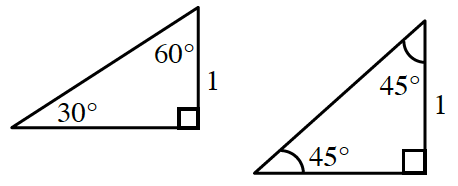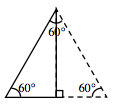Home > CCA2 > Chapter 7 > Lesson 7.1.2 > Problem7-15

7-15.Copy the triangles at right. Homework Help ✎

1. Label the missing sides with their exact lengths. That is, leave your answers in radical form.

2. The $30°-60°-90°$ triangle is sometimes called a half-equilateral triangle. Draw a picture to illustrate this, and explain how that fact can be used to help label the missing sides in part (a).

Refer to the Math Notes box for Lesson 7.1.2.

Reflect the $30°-60°-90°$ triangle across the long leg. This will help you find the hypotenuse.
Use the Pythagorean Theorem to find the length of the long leg.